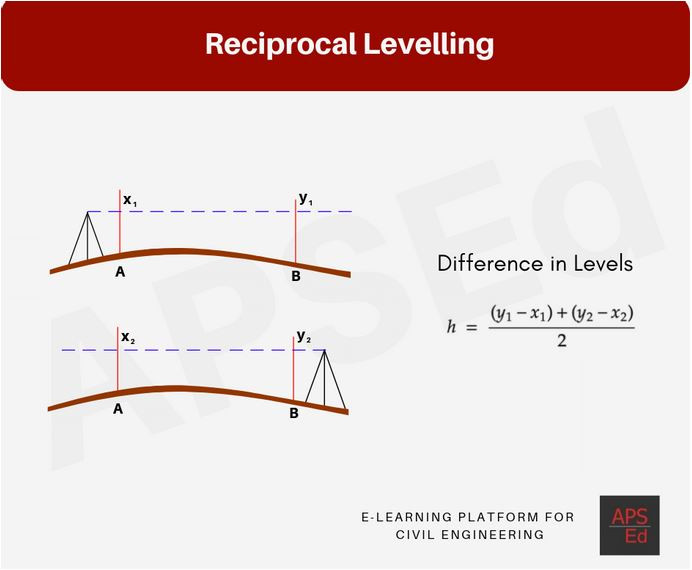Search
• APSEd

# Reciprocal Levelling | Curvature and Refraction Correction

Reciprocal levelling helps in compensating for the error due to curvature and refraction and also the line of collimation errors in surveying. It is one of the best methods to eliminate curvature and refraction errors.

In reciprocal levelling, the level is set up on both sides of the levels. Two sets of staff reading are taken. This helps in compensating for the error due to curvature and refraction & also the line of collimation errors in surveying.

## Finding Difference in Levels

Let us say A and B are two points.

• The instrument is set up near A and readings on the staff are noted (x1 and y1)

• The instrument is shifted near B and readings on staff are again noted (x2 and y2)

Assuming the curvature and refraction error combinedly be e.

The error occurred when the instrument is close to 'A' is considered equal to the error occurred when the instrument is close to 'B' because the instrument is shifted quickly and there is not much change in refraction error.

The difference in levels, h = (y1 - x1) = (y2 - x2)

Considering a combined errors e,

The difference in level h = ((y1 - e ) - x1)

It can also be written as, h = (y2 - (x2 - e))

On adding these two equations, 2h = (y1 - x1) + (y2 - x2)

So, h = 0.5((y1 - x1) + (y2 - x2))It is assumed that the atmospheric refraction remains at the same level when the instrument is shifted (Advisable to shift the instrument quickly!)

What about watching an 8 minutes video and understanding every bit of 'Reciprocal Levelling'?

## Curvature and Refraction Correction

### Curvature correction(Cc)

• When levelling is done in a large area, the curvature of Earth is considered.

• The horizontal line is not a level line, because of which the staff reading is more than expected, this is called curvature correction.

• The effect of curvature is to increase the staff reading that is the error is positive and so the correction is negative.Curvature Correction

The curvature correction(Cc) is mathematically expressed as,

Cc = d^2/2R

Where, d: the length of the sight or the distance from the instruments to the staff station in kilometres

R= the radius of the earth = 6371 kilometres

So, Cc = 0.0785d^2

### Refraction correction (Cr)

• The rays of light passing through the atmosphere of different density bend down. It results in this type of correction.

• The effect of refraction is 1/7th time the curvature correction but is of opposite nature. Hence the correction for refraction is additive to the staff reading.

Cr = 1/7 x Cc

So, Cr = 1/7 x 0.0785d^2 = 0.0112 x d^2